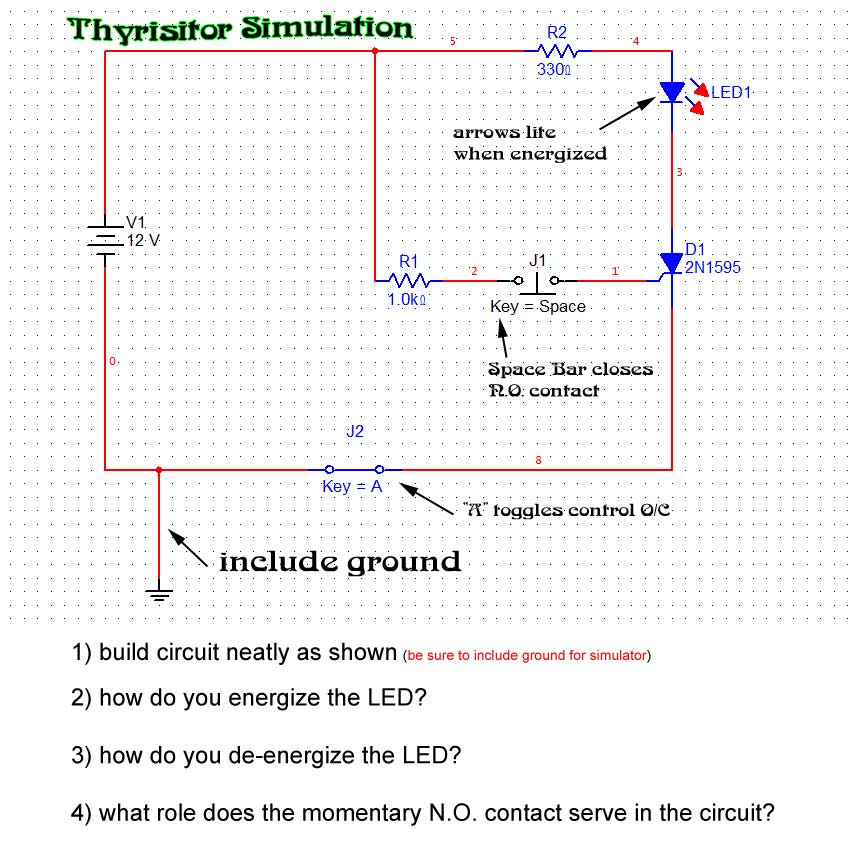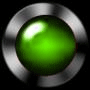circuit simulations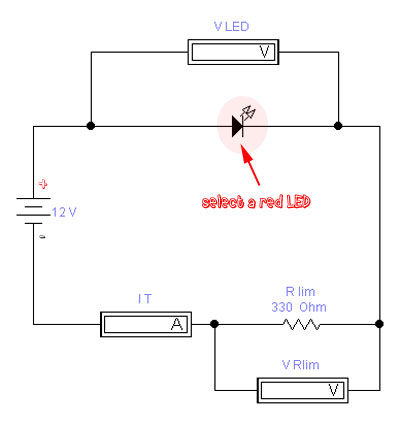1) Put a RED LED in series with a 12 VDC source and a 330 ohm limiting resistor. > predict and then measure VLED  and VRlim  and IT.  > What is the rated forward voltage of a RED LED? > Why does a BLUE LED require a greater forward voltage?HOW to read an oscilloscope read this over before proceeding Need a Calculator here it is 2) a) Build this half wave rectifier (one diode)  with 12VAC source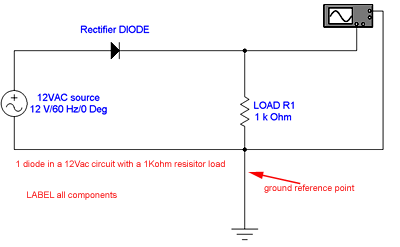in series with a 1 Kohm resistor , connect an oscilloscope to channel A across the resistor load. ***note a ground is needed now to act as reference for the oscilloscope set: time base to 5.00 ms/div set: Channel A to 10 v/div Calculate: f and Vmax across R1 > what type of electrical wave is generated at the output of the power supply?   HOW to read an oscilloscope read this over before proceeding Need a Calculator here it is 3) Build a bridge rectifier (shown below)  no capacitor at first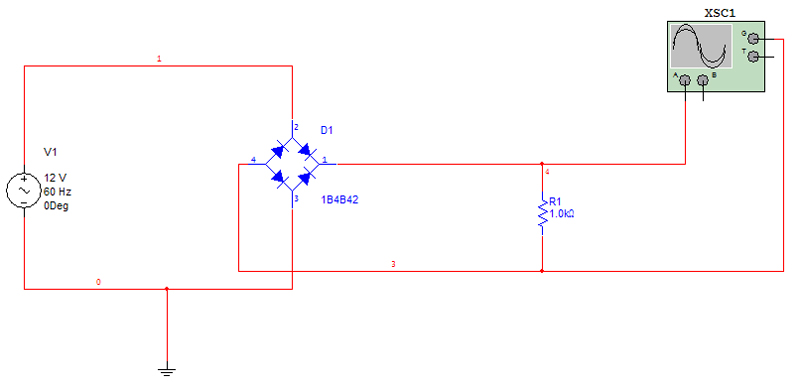set the following on the oscilloscope > time 5.00 ms/div this means that the trace is sweeping the screen at at a rate of 1 div per .005s > Channel A 5 V/div this means that the probe from channel A will deflect the trace 1 division for every 5V measured (ie 2 div =  10V) > add ground (reference) the ground reference is important for the simulator to measure a true voltage > set Trigger to A inputs to A trigger the trace to sweep this can be triggered other ways   a) add no filtering capacitor to oscilloscope in this instance Determine frequency = ___________ b) install 47uF cap in parallel to output of the power supply Need a Calculator here it is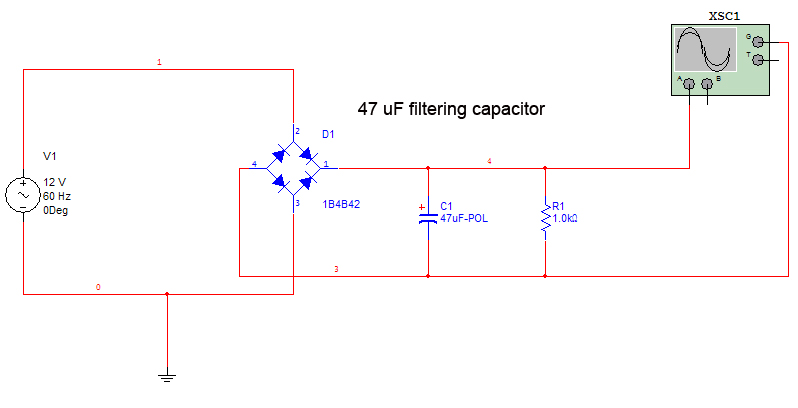Q- what happened to the wave characteristics after the capacitor was added?   c) install 1000uF cap in parallel to output of the power supply Need a Calculator here it is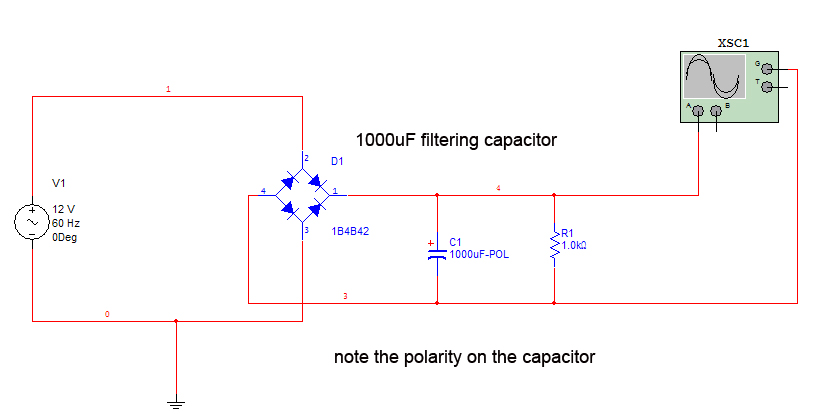Q- what happened to the wave characteristics? 4) Thyristors